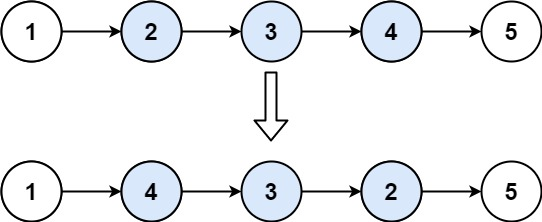# GeetCode Hub

Given the `head` of a singly linked list and two integers `left` and `right` where `left <= right`, reverse the nodes of the list from position `left` to position `right`, and return the reversed list.

Example 1:```Input: head = [1,2,3,4,5], left = 2, right = 4
Output: [1,4,3,2,5]
```

Example 2:

```Input: head = , left = 1, right = 1
Output: 
```

Constraints:

• The number of nodes in the list is `n`.
• `1 <= n <= 500`
• `-500 <= Node.val <= 500`
• `1 <= left <= right <= n`

Follow up: Could you do it in one pass?

/** * Definition for singly-linked list. * public class ListNode { * int val; * ListNode next; * ListNode() {} * ListNode(int val) { this.val = val; } * ListNode(int val, ListNode next) { this.val = val; this.next = next; } * } */ class Solution { public ListNode reverseBetween(ListNode head, int left, int right) { } }